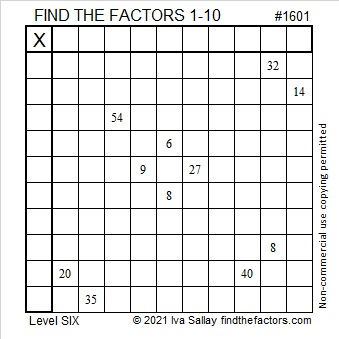# 1601 and Level 6

Contents

### Today’s Puzzle:

Remember to use logic for EVERY step when solving this puzzle. Guessing and checking will likely just frustrate you! It’s a level 6 puzzle, so it could be tricky.
Keep in mind:
1 and 2 are common factors of 6 and 8,
3 and 9 are common factors of 9 and 27,
4 & 8 are common factors of 32 and 8, and
4, 5, & 10 are common factors of 20 & 40.

As always, there is only one solution.### Factors of 1601:

• 1601 is a prime number.
• Prime factorization: 1601 is prime.
• 1601 has no exponents greater than 1 in its prime factorization, so √1601 cannot be simplified.
• The exponent in the prime factorization is 1. Adding one to that exponent we get (1 + 1) = 2. Therefore 1601 has exactly 2 factors.
• The factors of 1601 are outlined with their factor pair partners in the graphic below.

How do we know that 1601 is a prime number? If 1601 were not a prime number, then it would be divisible by at least one prime number less than or equal to √1601. Since 1601 cannot be divided evenly by 2, 3, 5, 7, 11, 13, 17, 19, 23, 29, 31, or 37, we know that 1601 is a prime number.### More about the Number 1601:

1601 is one more than a perfect square, so it is the sum of two squares:
40² + 1² = 1601.

1601 is the hypotenuse of a Pythagorean triple:
80-1599-1601, calculated from 2(40)(1), 40² – 1², 40² + 1².

Here’s another way we know that 1601 is a prime number: Since its last two digits divided by 4 leave a remainder of 1, and 40² + 1² = 1601 with 40 and 1 having no common prime factors, 1601 will be prime unless it is divisible by a prime number Pythagorean triple hypotenuse less than or equal to √1601. Since 1601 is not divisible by 5, 13, 17, 29, or 37, we know that 1601 is a prime number.

This site uses Akismet to reduce spam. Learn how your comment data is processed.